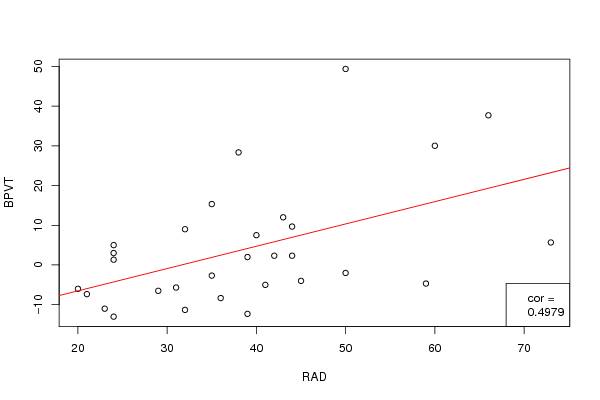## Free Statistics

of Irreproducible Research!

Author's title
Author*The author of this computation has been verified*
R Software Modulerwasp_Pregnancy and cognition.wasp
Title produced by softwareCorrelation
Date of computationSun, 29 May 2011 18:15:43 +0000
Cite this page as followsStatistical Computations at FreeStatistics.org, Office for Research Development and Education, URL https://freestatistics.org/blog/index.php?v=date/2011/May/29/t1306692682ppi8vbxr4xh79pv.htm/, Retrieved Thu, 06 Oct 2022 12:47:44 +0000
Statistical Computations at FreeStatistics.org, Office for Research Development and Education, URL https://freestatistics.org/blog/index.php?pk=122560, Retrieved Thu, 06 Oct 2022 12:47:44 +0000
QR Codes:Original text written by user:
IsPrivate?No (this computation is public)
User-defined keywords
Estimated Impact146
Family? (F = Feedback message, R = changed R code, M = changed R Module, P = changed Parameters, D = changed Data)
-     [Aston University Statistical Software] [Morning Sickness ...] [2009-11-16 16:26:06] [74be16979710d4c4e7c6647856088456]
- R     [Aston University Statistical Software] [Morning Sickness ...] [2009-11-16 17:22:16] [74be16979710d4c4e7c6647856088456]
-   P     [T-Tests] [Morning Sickness ...] [2010-11-09 11:12:43] [3fdd735c61ad38cbc9b3393dc997cdb7]
-           [T-Tests] [Week 6 compendium...] [2010-11-12 14:20:50] [88bba52b01540967ecde38774018061d]
- R  D        [T-Tests] [t test] [2011-05-29 17:38:02] [88bba52b01540967ecde38774018061d]
- RMPD          [Correlation] [correlation] [2011-05-29 18:08:41] [88bba52b01540967ecde38774018061d]
- R               [Correlation] [spearman] [2011-05-29 18:11:55] [88bba52b01540967ecde38774018061d]
-                     [Correlation] [spearman] [2011-05-29 18:15:43] [add39d12cbc73ea169e24b6abf6153f7] [Current]
Feedback Forum

Post a new message
Dataseries X:
29
21
50
23
35
36
32
44
42
39
20
73
38
43
50
32
24
24
59
41
24
44
66
40
24
31
45
60
35
39
Dataseries Y:
-6.5
-7.33
49.33
-11
-2.67
-8.33
9
9.67
2.33
-12.3
-6
5.67
28.33
12
-2
-11.3
1.33
3
-4.67
-5
-13
2.33
37.67
7.5
5
-5.67
-4
30
15.33
2

 Summary of computational transaction Raw Input view raw input (R code) Raw Output view raw output of R engine Computing time 1 seconds R Server 'Herman Ole Andreas Wold' @ www.yougetit.org

\begin{tabular}{lllllllll}
\hline
Summary of computational transaction \tabularnewline
Raw Input & view raw input (R code)  \tabularnewline
Raw Output & view raw output of R engine  \tabularnewline
Computing time & 1 seconds \tabularnewline
R Server & 'Herman Ole Andreas Wold' @ www.yougetit.org \tabularnewline
\hline
\end{tabular}
%Source: https://freestatistics.org/blog/index.php?pk=122560&T=0

[TABLE]
[ROW][C]Summary of computational transaction[/C][/ROW]
[ROW][C]Raw Input[/C][C]view raw input (R code) [/C][/ROW]
[ROW][C]Raw Output[/C][C]view raw output of R engine [/C][/ROW]
[ROW][C]Computing time[/C][C]1 seconds[/C][/ROW]
[ROW][C]R Server[/C][C]'Herman Ole Andreas Wold' @ www.yougetit.org[/C][/ROW]
[/TABLE]
Source: https://freestatistics.org/blog/index.php?pk=122560&T=0

Globally Unique Identifier (entire table): ba.freestatistics.org/blog/index.php?pk=122560&T=0

As an alternative you can also use a QR Code:

The GUIDs for individual cells are displayed in the table below:

 Summary of computational transaction Raw Input view raw input (R code) Raw Output view raw output of R engine Computing time 1 seconds R Server 'Herman Ole Andreas Wold' @ www.yougetit.org

 Shapiro Test (RAD) Statistic W Probability Link 0.9395 0.0882 Shapiro-Wilk Test

\begin{tabular}{lllllllll}
\hline
Shapiro Test (RAD) \tabularnewline
Statistic W & Probability & Link \tabularnewline
0.9395 & 0.0882 & Shapiro-Wilk Test \tabularnewline
\hline
\end{tabular}
%Source: https://freestatistics.org/blog/index.php?pk=122560&T=1

[TABLE]
[ROW][C]0.9395[/C][C]0.0882[/C][C]Shapiro-Wilk Test[/C][/ROW]
[/TABLE]
Source: https://freestatistics.org/blog/index.php?pk=122560&T=1

Globally Unique Identifier (entire table): ba.freestatistics.org/blog/index.php?pk=122560&T=1

As an alternative you can also use a QR Code:

The GUIDs for individual cells are displayed in the table below:

 Shapiro Test (RAD) Statistic W Probability Link 0.9395 0.0882 Shapiro-Wilk Test

 Shapiro Test (BPVT) Statistic W probability Link 0.854 8e-04 Shapiro-Wilk Test

\begin{tabular}{lllllllll}
\hline
Shapiro Test (BPVT) \tabularnewline
Statistic W & probability & Link \tabularnewline
0.854 & 8e-04 & Shapiro-Wilk Test \tabularnewline
\hline
\end{tabular}
%Source: https://freestatistics.org/blog/index.php?pk=122560&T=2

[TABLE]
[ROW][C]Shapiro Test (BPVT)[/C][/ROW]
[ROW][C]0.854[/C][C]8e-04[/C][C]Shapiro-Wilk Test[/C][/ROW]
[/TABLE]
Source: https://freestatistics.org/blog/index.php?pk=122560&T=2

Globally Unique Identifier (entire table): ba.freestatistics.org/blog/index.php?pk=122560&T=2

As an alternative you can also use a QR Code:

The GUIDs for individual cells are displayed in the table below:

 Shapiro Test (BPVT) Statistic W probability Link 0.854 8e-04 Shapiro-Wilk Test

 Correlation Test X-Y Statistic Value probability Method alternative rho 0.514 0.0037 Spearman's rank correlation rho two.sided

\begin{tabular}{lllllllll}
\hline
Correlation Test X-Y \tabularnewline
Statistic & Value & probability & Method & alternative \tabularnewline
rho & 0.514 & 0.0037 & Spearman's rank correlation rho & two.sided \tabularnewline
\hline
\end{tabular}
%Source: https://freestatistics.org/blog/index.php?pk=122560&T=3

[TABLE]
[ROW][C]Correlation Test X-Y[/C][/ROW]
[ROW][C]Statistic[/C][C]Value[/C][C]probability[/C][C]Method[/C][C]alternative[/C][/ROW]
[ROW][C]rho[/C][C]0.514[/C][C]0.0037[/C][C]Spearman's rank correlation rho[/C][C]two.sided[/C][/ROW]
[/TABLE]
Source: https://freestatistics.org/blog/index.php?pk=122560&T=3

Globally Unique Identifier (entire table): ba.freestatistics.org/blog/index.php?pk=122560&T=3

As an alternative you can also use a QR Code:

The GUIDs for individual cells are displayed in the table below:

 Correlation Test X-Y Statistic Value probability Method alternative rho 0.514 0.0037 Spearman's rank correlation rho two.sidedPNG link Postscript link PDF link

Parameters (Session):
Parameters (R input):
par1 = spearman ; par2 = two.sided ;
R code (references can be found in the software module):
bitmap(file='test1.png')plot(x,y, xlab=xlab, ylab=ylab)abline(coef=(lm(y ~ x))$coeff, col='red')ctp<-cor.test(x, y, use='pair', method='pearson')legend('bottomright', c('cor =', as.character(round(ctp$estimate, digits=4))))dev.off()(sw1<-shapiro.test(x))(sw2<-shapiro.test(y))(cxy<-cor.test(x, y, method=par1))load(file='createtable')a<-table.start()a<-table.row.start(a)a<-table.element(a,paste('Shapiro Test (', xlab,')',sep=''),3,TRUE)a<-table.row.end(a)a<-table.row.start(a)a<-table.element(a,'Statistic W',header=TRUE)a<-table.element(a,'Probability',header=TRUE)a<-table.element(a,'Link',header=TRUE)a<-table.row.end(a)a<-table.row.start(a)a<-table.element(a,round(sw1$statistic, digits=4))a<-table.element(a,round(sw1$p.value, digits=4))a<-table.element(a,hyperlink('http://en.wikipedia.org/wiki/Shapiro%E2%80%93Wilk_test','Shapiro-Wilk Test','Shapiro-Wilk Test'))a<-table.row.end(a)a<-table.end(a)table.save(a,file='mytable.tab')a<-table.start()a<-table.row.start(a)a<-table.element(a, paste('Shapiro Test (', ylab,')',sep=''),3,TRUE)a<-table.row.end(a)a<-table.row.start(a)a<-table.element(a,'Statistic W',header=TRUE)a<-table.element(a,'probability',header=TRUE)a<-table.element(a,'Link',header=TRUE)a<-table.row.end(a)a<-table.row.start(a)a<-table.element(a,round(sw2$statistic, digits=4))a<-table.element(a,round(sw2$p.value, digits=4))a<-table.element(a,hyperlink('http://en.wikipedia.org/wiki/Shapiro%E2%80%93Wilk_test','Shapiro-Wilk Test','Shapiro-Wilk Test'))a<-table.row.end(a)a<-table.end(a)table.save(a,file='mytable1.tab')a<-table.start()a<-table.row.start(a)a<-table.element(a,'Correlation Test X-Y',5,TRUE)a<-table.row.end(a)a<-table.row.start(a)a<-table.element(a,'Statistic',header=TRUE)a<-table.element(a,'Value',header=TRUE)a<-table.element(a,'probability',header=TRUE)a<-table.element(a,'Method',header=TRUE)a<-table.element(a,'alternative',header=TRUE)a<-table.row.end(a)a<-table.row.start(a)a<-table.element(a,names(cxy$estimate))a<-table.element(a,round(cxy$estimate, digits=4))a<-table.element(a,round(cxy$p.value, digits=4))a<-table.element(a,cxy$method)a<-table.element(a,cxy\$alternative)a<-table.row.end(a)a<-table.end(a)table.save(a,file='mytable2.tab')